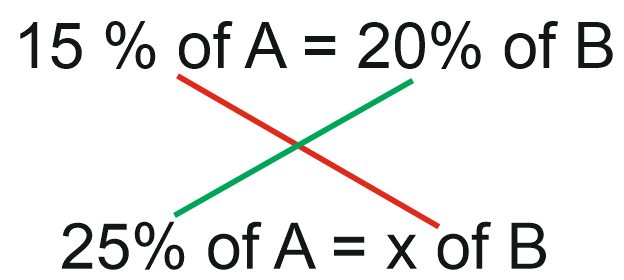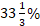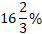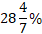• Save

# Percentage Shortcut Tricks For Bank PO Exams

2 months ago 5.2K ViewsPercent is an important chapter of mathematics and its questions have been asked in the Competition Examination for many years. If you are preparing for SSC and banking exams then you should come to solve the percentage questions.

Once you understand the percentage, you can get good marks in the exam. | In this blog, I am going to tell you a simple trick of percentage with the help of which you can easily solve the percentage questions.

## Percentage Shortcut Tricks for Competitive Exams:

What is the percentage?

In mathematics, a percentage is a number or ratio expressed as a fraction of 100.

For example:

X% in fraction form is X/100.

Example.1. If 15% of A is equal to 20% of B, then 25% of A is equal to what percentage of B?

Short tricks

15% of A = 20% of B

25% Of A = x of BX = (20×25)/15 = 100/3 =Example.2. The monthly income of a person is Rs 8000. If his income is increased by 20%, What will be his new monthly income?

Short tricks

New income = 120% of 8000 = (12/100)×8000 = 9600.

Example.3. If income of Ravi is 20% more than that of Ram, then income of Ram is how much percent less than that of Ravi?

Let Ram’s income be = 100

Then, Ravi’s income = 120

⸫ Required percentage = (20/120)×(100) = 50/3 =Example.4. If the price of a commodity be raised by 40%, by how much percent a householder reduces his consumption of that commodity, so as not to increase his expenditure?

Short trick

Let initial price be 100

Price after increase = 140

⸫ Required reduction = (40/140)×100 =Example.5. A student has to score 30% marks to get through. If he gets 30 marks and fails by 30 marks, then find the maximum marks set for the examination.

Short tricks

Let the maximum marks be X

According to the question,

30X/100 = (30+30) → 30X/100 = 60 → X = 200

Example.6. In an examination, 20% of total number of students failed in History, 15% of total number of students failed in Hindi and 5% of total number of students failed in both. Find the percentage of students, who passed in both the subjects.

Let total number of students be 100

Then, students failed in History only = 20 – 5 = 15

And Students failed in Hindi only = 15 – 5 = 10

⸫ Total number of failed students = 15+10+5=30

⸫ Number of passed students in both the subject = 100-30= 70

⸫ Required percentage = 70%

## Practice excise of percentage questions:

Q.1.What the price of fans was reduced by 20%, the number of fans sold is increased by 40%. What was the effect on the sales in rupees?

(A) 12% decrease

(B)  12% increase

(C)  30% increase

(D)  40% decrease

Ans .   B

Q.2. A screwdriver and a hammer cost the same now. If the price of a screwdriver in increased by 5% and that of the hammer by 3%, how much more will it cost to buy 3 screwdriver and 3 hammers?

(A) 4%

(B) 5%

(C) 8%

(D) 24%

Ans .   A

Q.3. The population of a town is 12000. If the male population increases by 6% every and that of female by 8% then the total population at the end of the year become 12800. Find the number of males in the town.

(A) 8000

(B) 7500

(C) 7200

(D) 6500

Ans .  A

Q.4. A student was asked to measure the length and breadth of a rectangle. By mistake, he measured the length 20% less and the breadth 10% more. If its original area is 200 sq cm, then find the area after this measurement

(A) 176 sq cm

(B) 206 sq cm

(C) 226 sq cm

(D) 316 sq cm

Ans .  A

Q.5. Shohan spends 23% of an amount of money on an insurance policy, 33% on food, 19% on children’s education and 16% on recreation. He deposits the remaining amount of Rs 504 in bank. How much total amount of Rs 504 in bank. How much total amount does he spend on food and insurance policy together?

(A) 3200

(B) 3126

(C) 3136

(D) 3048

Ans .  C

If you want to practice more questions related to Percentage then you can go to the link provided below.

In this post I have made every effort to explain the Percentage in a simple way, even if you are having problems in any manner or question, you can Ask me by writing in the comment box below.